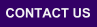Mplus
Tuesday
April 20, 2021Mplus Mplus at a GlanceGeneral DescriptionMplus Programs Pricing Version History System Requirements Platforms FAQ
 Analyses/ResearchMplus Examples Papers References
 Search

Chapter 1: Linear regression analysis

Download all Chapter 1 tables

Table View output Download input Download data
Table 1.2, Part 1, p. 12: Linear regression with one covariate 1.2 (Part 1) 1.2 (Part 1) .inp dropout.dat
Table 1.2, Part 2, p. 13: Linear regression with one covariate and outliers 1.2 (Part 2) 1.2 (Part 2) .inp dropout.dat
Table 1.4, pp. 24-27: Linear regression with two covariates 1.4 1.4 .inp dropout.dat
Table 1.8, Part 1, pp. 29-31: Regression with a randomized intervention using treatment-baseline interaction 1.8 (Part 1) 1.8 (Part 1) .inp Data are unavailable
Table 1.8, Part 2, p. 31: Regression with a randomized intervention using treatment-baseline interaction and LOOP PLOT of moderation 1.8 (Part 2) 1.8 (Part 2) .inp Data are unavailable
Table 1.8, Part 3, p. 33: Regression with a randomized intervention using treatment-baseline interaction and LOOP PLOT of conditional means 1.8 (Part 3) 1.8 (Part 3) .inp Data are unavailable
Table 1.11, pp. 38-39: Two-group regression analysis of randomized intervention using treatment-baseline interaction 1.11 1.11 .inp Data are unavailable
Table 1.13, pp. 42-43: Two-group regression analysis of randomized intervention using treatment-baseline interaction, bringing covariates into the model 1.13 1.13 .inp Data are unavailable
Table 1.15, pp. 47-48: Linear regression with heteroscedasticity modeling 1.15 1.15 .inp dropout.dat
Table 1.18, p. 51: Random coefficient regression with heteroscedasticity 1.18 1.18 .inp dropout.dat
Table 1.19, Part 1, pp. 52-53: Random coefficient regression with heteroscedasticity and moderators 1.19 (Part 1) 1.19 (Part 1) .inp dropout.dat
Table 1.19, Part 2, pp. 52-53: Regression ignoring heteroscedasticity using interaction terms 1.19 (Part 2) 1.19 (Part 2) .inp dropout.dat
Table 1.21, p. 54: Random coefficient regression with conditional mean plots 1.21 1.21 .inp dropout.dat